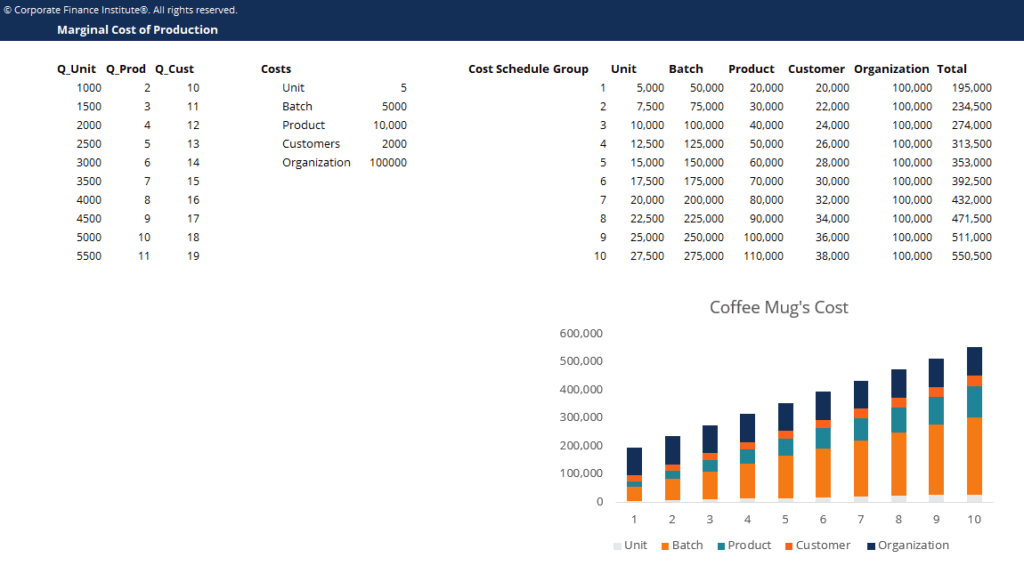# Marginal Cost of Production Template

## Marginal Cost of Production Template

This marginal cost of production template will show you how to calculate different types of marginal costs.

This is what the marginal cost of production template looks like:### Download the Free Template

Enter your name and email in the form below and download the free template now!

### Marginal Cost Production Template

Download the free Excel template now to advance your finance knowledge!

The Marginal Cost of production is the cost to provide one additional unit of a product or service. It is a fundamental principle to derive economically optimal decisions and an important aspect of managerial accounting and financial analysis. It can be calculated as:

Marginal Cost = Change in Cost / Change in Quantity

If a company’s total cost of production is defined as:

TC = FC + (VC x Q)

Then its marginal cost is the first order derivative of the total cost function. In this case, the marginal cost is directly equal to its variable costs.

MC = dTC / dQ = VC

Where:

TC: Total Cost

FC: Fixed Cost

Q: Quantity

VC: Variable Cost

MC: Marginal Cost

### More Free Templates

For more resources, check out our business templates library to download numerous free Excel modeling, PowerPoint presentation and Word document templates.

• Excel Modeling Templates
• PowerPoint Presentation Templates
• Transaction Document Templates

### Financial Analyst Certification

Become a certified Financial Modeling and Valuation Analyst (FMVA)® by completing CFI’s online financial modeling classes and training program!